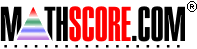Math Practice Online > free > lessons > Florida > 6th grade > Single Variable Equations

## Single Variable Equations

Solve single variable equations in one step.

 Sample Problems for Single Variable Equations Lesson for Single Variable Equations

### This topic aligns to the following state standards

Grade 6: Alg 4. Uses pictures, models, manipulatives or other strategies to solve simple one-step linear equations with rational solutions.
Grade 7: Alg 4. Uses pictures, models, manipulatives or other strategies to solve one-step and simple multi- step linear equations.
Grade 8: Alg 3. Solves single- and multiple-step linear equations and inequalities in concrete or abstract form.

Copyright Accurate Learning Systems Corporation 2008.